• Slides: 37QUADRATIC EQUATIONS DEFINITION OF QUADRATIC EXPRESSION, EQUATION & ITS ROOTSQUADRATIC EQUATIONS Quadratic Expression: We know that quadratic means 2+bx+c, degree ‘ 2’ A polynomial of the form ax where a, b, c are complex numbers and a 0 variable ‘x’. Example: is called a quadratic expression in the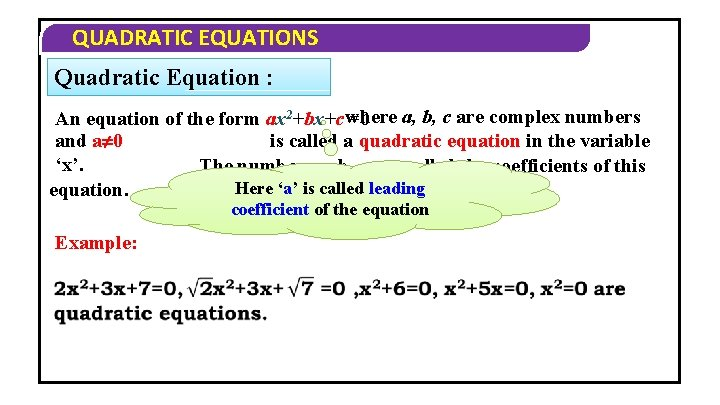QUADRATIC EQUATIONS Quadratic Equation : a, b, c are complex numbers An equation of the form ax 2+bx+c where =0 and a 0 is called a quadratic equation in the variable ‘x’. The numbers a, b, c are called the coefficients of this Here ‘a’ is called leading equation. coefficient of the equation Example: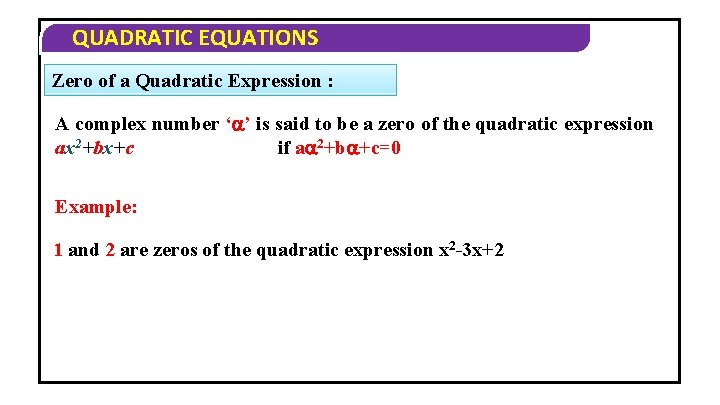QUADRATIC EQUATIONS Zero of a Quadratic Expression : A complex number ‘ ’ is said to be a zero of the quadratic expression if a 2+b +c=0 ax 2+bx+c Example: 1 and 2 are zeros of the quadratic expression x 2 -3 x+2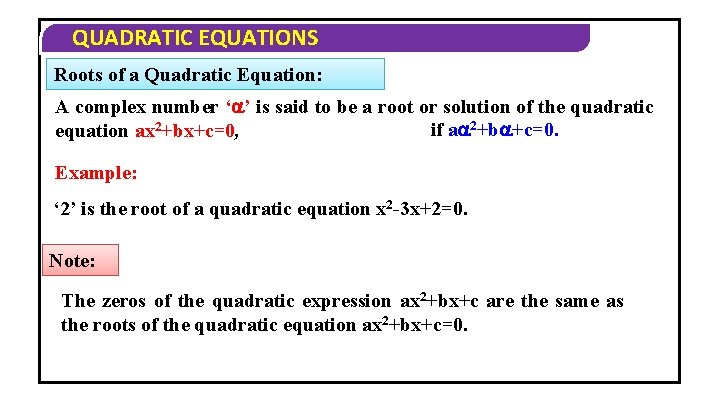QUADRATIC EQUATIONS Roots of a Quadratic Equation: A complex number ‘ ’ is said to be a root or solution of the quadratic if a 2+b +c=0. equation ax 2+bx+c=0, Example: ‘ 2’ is the root of a quadratic equation x 2 -3 x+2=0. Note: The zeros of the quadratic expression ax 2+bx+c are the same as the roots of the quadratic equation ax 2+bx+c=0.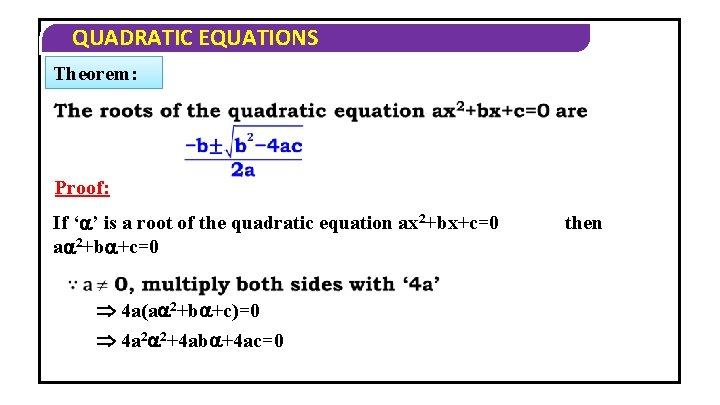QUADRATIC EQUATIONS Theorem: Proof: If ‘ ’ is a root of the quadratic equation ax 2+bx+c=0 a 2+b +c=0 4 a(a 2+b +c)=0 4 a 2 2+4 ab +4 ac=0 then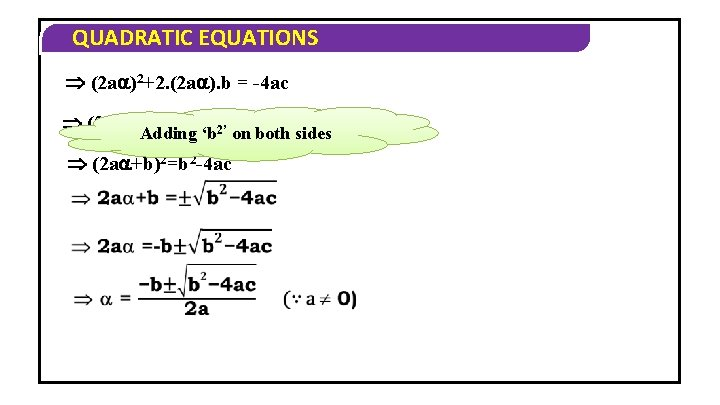QUADRATIC EQUATIONS (2 a )2+2. (2 a ). b = -4 ac 2=b 2 -4 ac (2 a )2 Adding +2. (2 a ). b+b 2’ ‘b on both sides (2 a +b)2=b 2 -4 acQUADRATIC EQUATIONS Note: 1) A quadratic equation ax 2+bx+c=0 has two roots (real or complex, not necessarily distinct) 2) If both roots of the quadratic equation are equal to ,QUADRATIC EQUATIONS VERY SHORT ANSWER QUESTIONS 1. Find the roots of the equation 3 x 2+2 x-5=0 Solution Here a=3, b=2, c=-5 The roots of the given equation are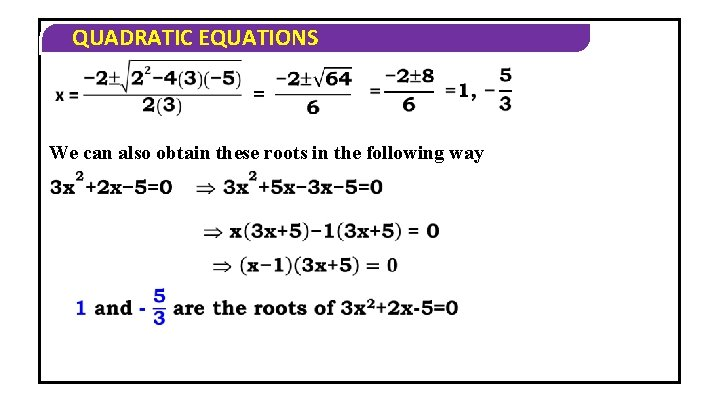QUADRATIC EQUATIONS We can also obtain these roots in the following way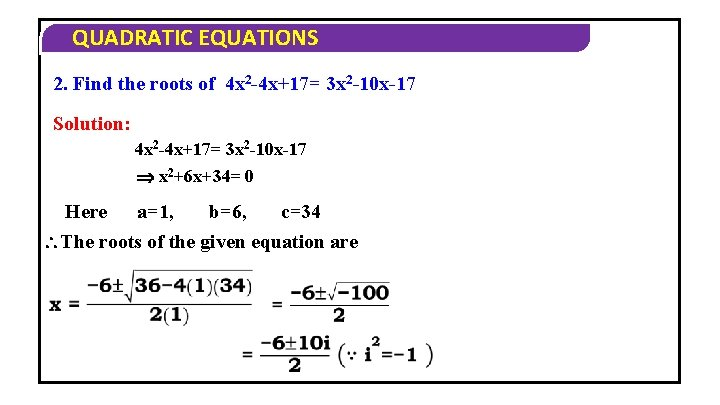QUADRATIC EQUATIONS 2. Find the roots of 4 x 2 -4 x+17= 3 x 2 -10 x-17 Solution: 4 x 2 -4 x+17= 3 x 2 -10 x-17 x 2+6 x+34= 0 Here a=1, b=6, c=34 The roots of the given equation areQUADRATIC EQUATIONS = -3 +5 i, -3 -5 i Hence, the roots of the given equation are -3+5 i, -3 -5 i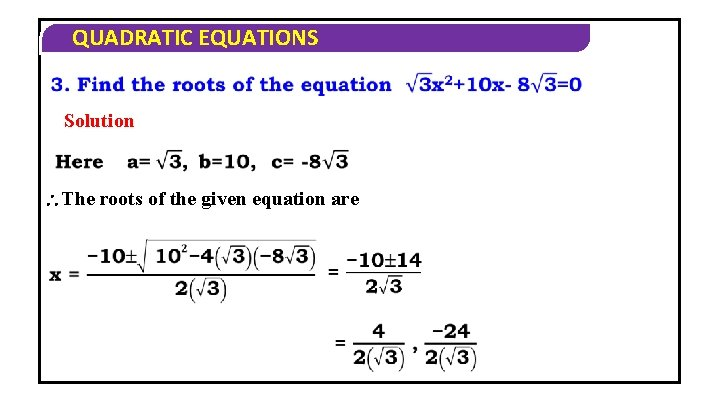QUADRATIC EQUATIONS Solution The roots of the given equation are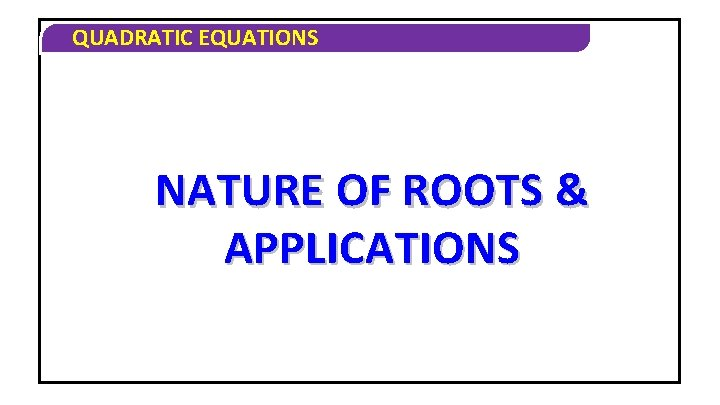QUADRATIC EQUATIONS NATURE OF ROOTS & APPLICATIONS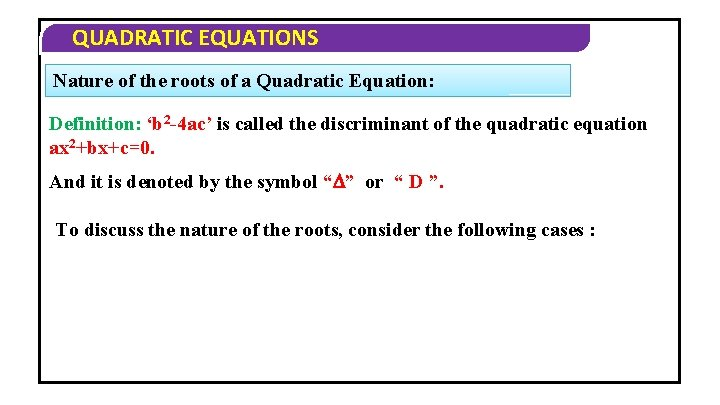QUADRATIC EQUATIONS Nature of the roots of a Quadratic Equation: Definition: ‘b 2 -4 ac’ is called the discriminant of the quadratic equation ax 2+bx+c=0. And it is denoted by the symbol “ ” or “ D ”. To discuss the nature of the roots, consider the following cases :QUADRATIC EQUATIONS Let , be the roots of the quadratic equation ax 2+bx+c=0 Case I: If a, b, c are real numbers, then (i) >0 and are real and distinct (iii) <0 and are non-real complex numbers conjugate to each other.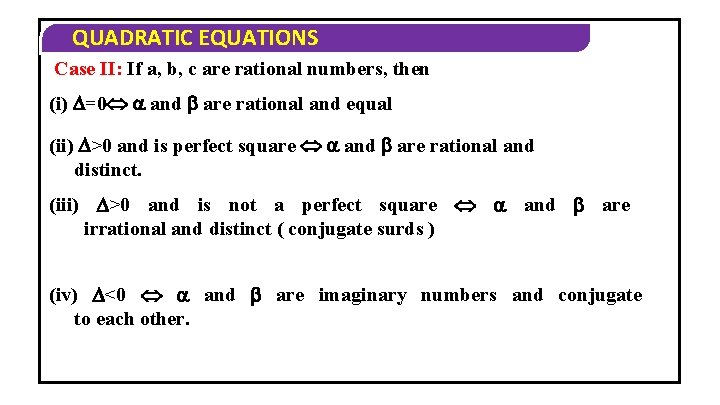QUADRATIC EQUATIONS Case II: If a, b, c are rational numbers, then (i) =0 and are rational and equal (ii) >0 and is perfect square and are rational and distinct. (iii) >0 and is not a perfect square and are irrational and distinct ( conjugate surds ) (iv) <0 and are imaginary numbers and conjugate to each other.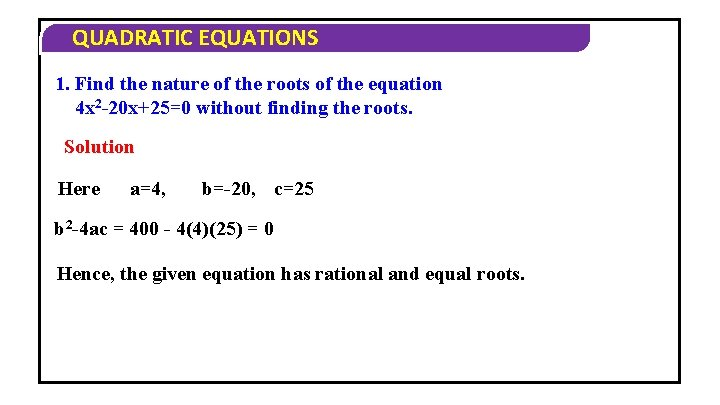QUADRATIC EQUATIONS 1. Find the nature of the roots of the equation 4 x 2 -20 x+25=0 without finding the roots. Solution Here a=4, b=-20, c=25 b 2 -4 ac = 400 - 4(4)(25) = 0 Hence, the given equation has rational and equal roots.QUADRATIC EQUATIONS 2. Show that the equation 2 x 2 -6 x+7=0 has no real root. Solution Here a=2, b=-6, c=7 b 2 -4 ac = (-6)2 - 4(2)(7) = -20 <0 The given equation has no real root.QUADRATIC EQUATIONS 3. Show that the roots of (x-a)(x-b)=h 2 are always real. Solution Given equation can be written as x 2 -(a+b)x+ab-h 2=0 comparing with Ax 2+Bx+C=0 Here A=1, B=-(a+b), C=ab-h 2 B 2 -4 AC = (a+b)2 - 4(1)(ab-h 2) = (a+b)2 – 4 ab+4 h 2 = (a-b)2 + 4 h 2 0 The roots of given equation are always real.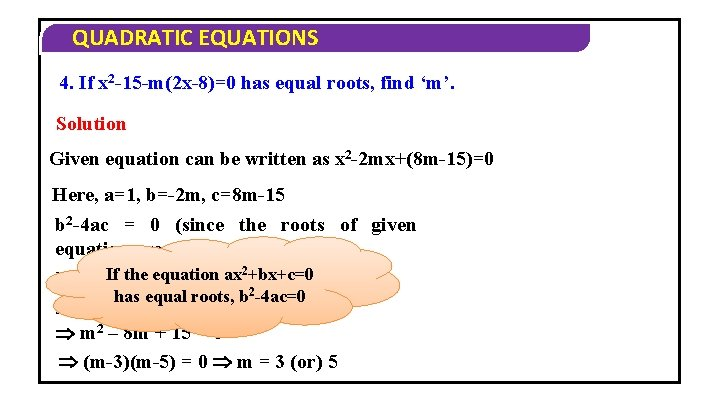QUADRATIC EQUATIONS 4. If x 2 -15 -m(2 x-8)=0 has equal roots, find ‘m’. Solution Given equation can be written as x 2 -2 mx+(8 m-15)=0 Here, a=1, b=-2 m, c=8 m-15 b 2 -4 ac = 0 (since the roots of given equation are equal) 2 – 4(1)(8 m-15) equation ax +bx+c=0= 0 i. e. , If the (-2 m) has equal roots, b 2 -4 ac=0 2 4 m – 32 m + 60 = 0 m 2 – 8 m + 15 = 0 (m-3)(m-5) = 0 m = 3 (or) 5 2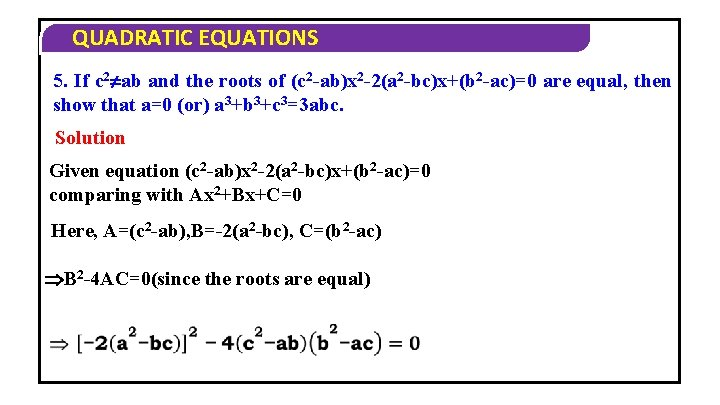QUADRATIC EQUATIONS 5. If c 2 ab and the roots of (c 2 -ab)x 2 -2(a 2 -bc)x+(b 2 -ac)=0 are equal, then show that a=0 (or) a 3+b 3+c 3=3 abc. Solution Given equation (c 2 -ab)x 2 -2(a 2 -bc)x+(b 2 -ac)=0 comparing with Ax 2+Bx+C=0 Here, A=(c 2 -ab), B=-2(a 2 -bc), C=(b 2 -ac) B 2 -4 AC=0(since the roots are equal)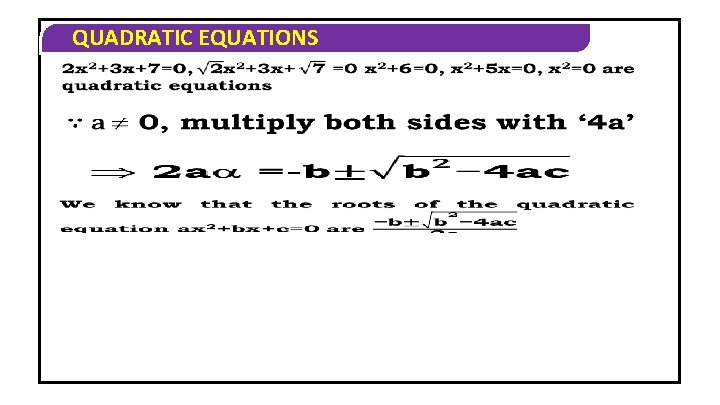QUADRATIC EQUATIONS PROBLEMS ON SUM & PRODUCT OF THE ROOTS OF Q. EQUADRATIC EQUATIONS Note 1. If and are the roots of ax 2+bx+c=0 then + =-b/a and =c/a Proof Since the roots of ax 2+bx+c=0 areQUADRATIC EQUATIONS Note 2. If and are the roots of ax 2+bx+c=0 then ax 2+bx+c=a(x- ) Proof + =-b/a =c/a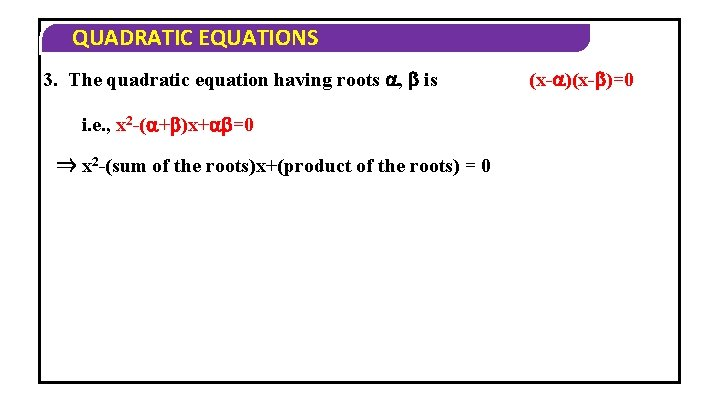QUADRATIC EQUATIONS 3. The quadratic equation having roots , is i. e. , x 2 -( + )x+ =0 ⇒ x 2 -(sum of the roots)x+(product of the roots) = 0 (x- )=0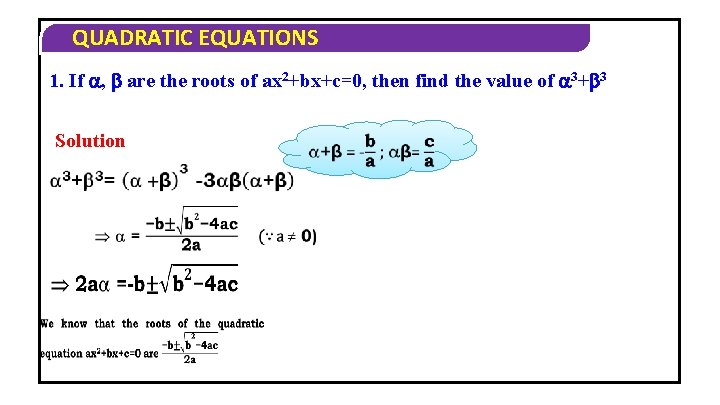QUADRATIC EQUATIONS 1. If , are the roots of ax 2+bx+c=0, then find the value of 3+ 3 Solution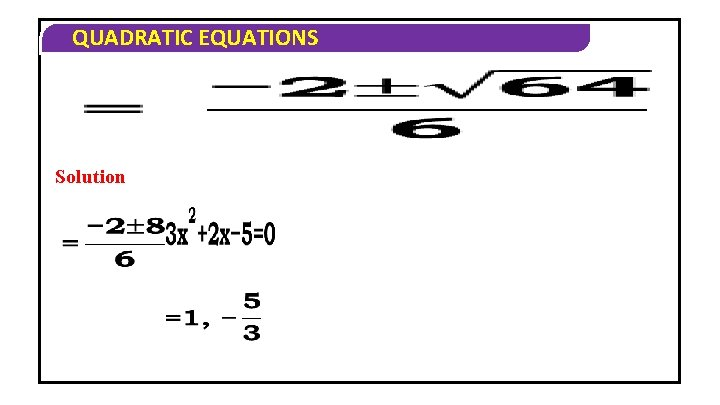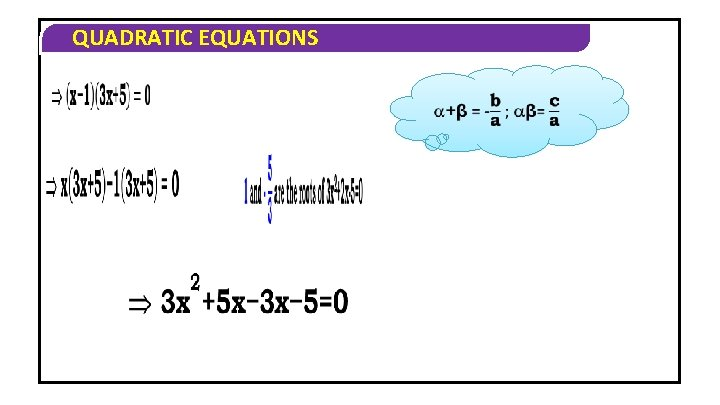QUADRATIC EQUATIONS 3. If , are the roots of ax 2+bx+c=0, then find the value of 4 7+ 7 4 SolutionQUADRATIC EQUATIONS Solution The quadratic equation having roots , is x 2( + )x+ =0QUADRATIC EQUATIONS =4, =1 The quadratic equation having roots , is x 2( + )x+ =0QUADRATIC EQUATIONS Thank you…